We all mark the Date table as a date table, because we know it is a best practice. But why? What happens if you do not mark the Date table as such?

In order to understand the importance of marking the Date table, we can use a simple year-to-date calculation to refresh our understanding of DAX evaluation contexts:

```Sales YTD =
CALCULATE (
[Sales Amount],
DATESYTD ( 'Date'[Date] )
)
```

The measure relies on the DATESYTD function. DATESYTD returns all the dates in the range between the first of January and the last visible date in the filter context. DATESYTD is the very same as the following table expression:

```VAR LastVisibleDate = MAX ( 'Date'[Date] )
RETURN
FILTER (
ALL ( 'Date'[Date] ),
'Date'[Date] >= DATE ( YEAR ( LastVisibleDate ), 1, 1 )
&& 'Date'[Date] <= LastVisibleDate
)
```

Therefore, DATESYTD returns a table containing dates. To be more specific, it contains some of the values of the Date[Date] column. When used in a report, the measure works well as you can see below.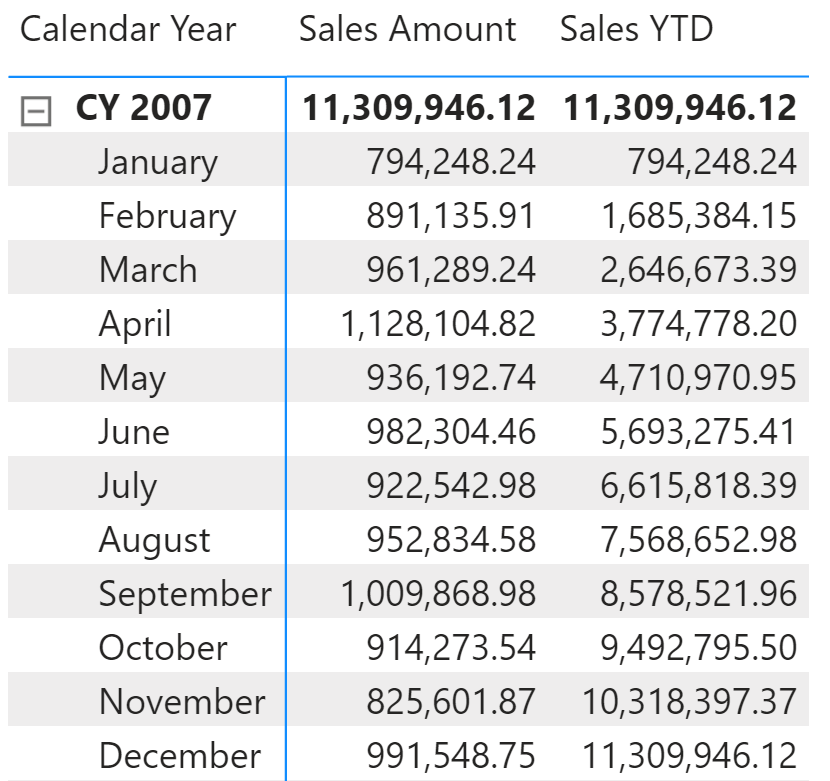Even though this probably does not surprise you, in fact it should. The measure – as it is written – should not work. The reason why it works is because the relationship between Sales and Date is using the Sales[Order Date] column. Sales[Order Date] has a Date data type. If we use an integer to relate the two tables instead of using a Date column, the formula stops working. We now change the relationship using the integer column Sales[OrderDateKey] instead of the Sales[Order Date].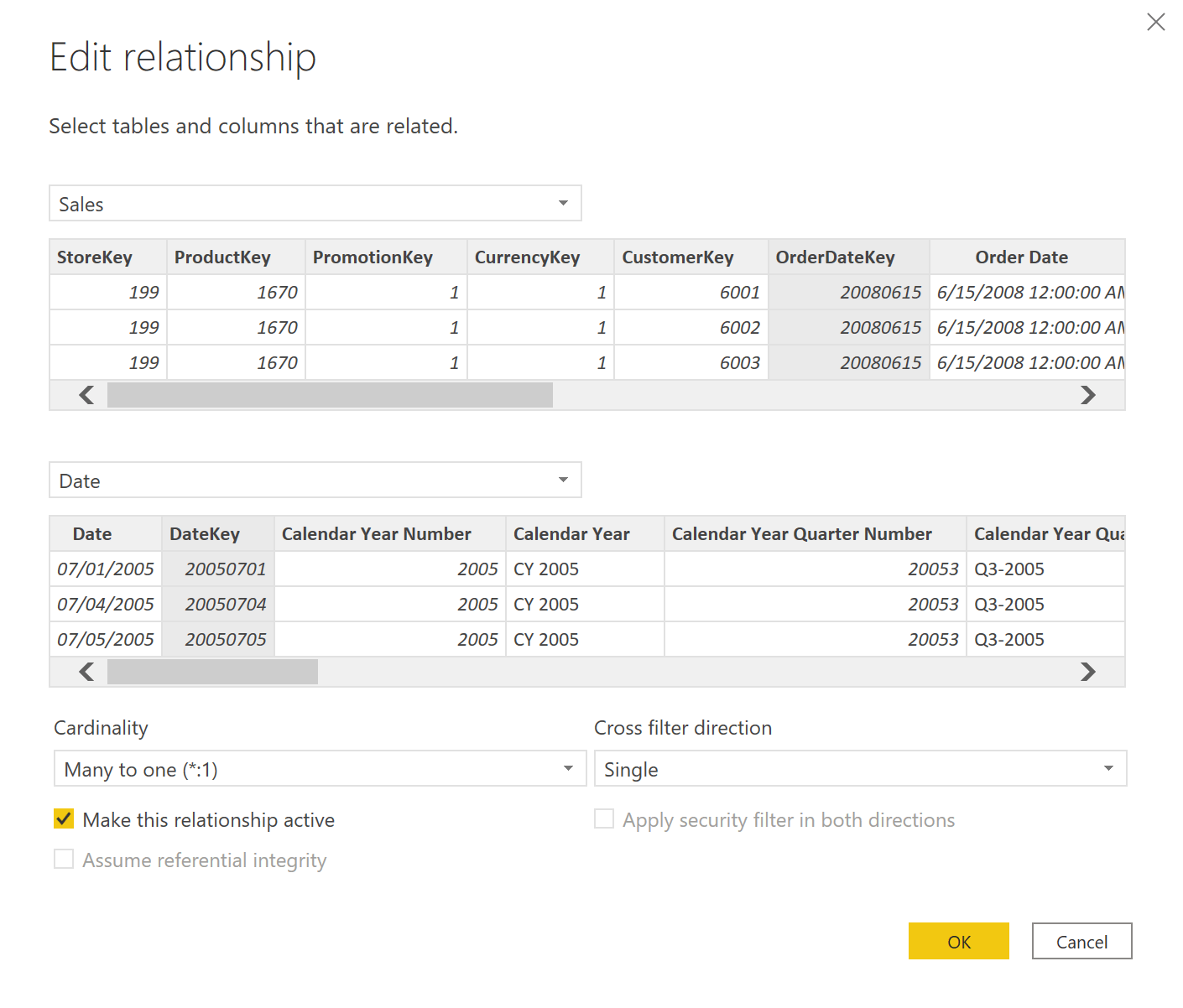The following figure shows the same matrix with the new relationship in place. The Sales YTD measure does no longer work.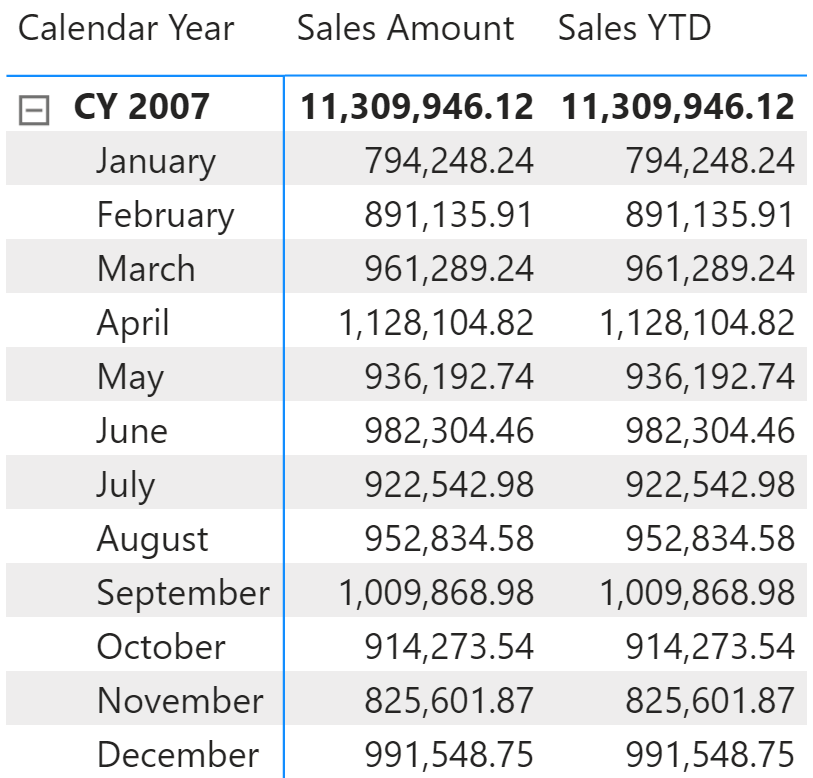Let us discover the reason. Focus on the cell of April, where both Sales Amount and Sales YTD return 1,128,104.92. This is the filter context under which Sales YTD is evaluated:

```'Date'[Calendar Year] = "CY 2007",
'Date'[Month] = "April"
```

Therefore, the evaluation of Sales YTD executes the following steps:

```CALCULATE (
[Sales YTD],
'Date'[Calendar Year] = "CY 2007",
'Date'[Month] = "April"
)
```

If you expand the definition of Sales YTD, this becomes:

```CALCULATE (
CALCULATE (
[Sales Amount],
DATESYTD ( 'Date'[Date] )
),
'Date'[Calendar Year] = "CY 2007",
'Date'[Month] = "April"
)
```

Now, what is the filter context under which Sales Amount is evaluated? We know that DATESYTD returns values from the column Date[Date]. Therefore, the filter produced by DATESYTD only filters Date[Date]. It does not overwrite the outer filter on Date[Calendar Year], nor the one on Date[Month]. Therefore, the filter context that evaluates Sales Amount becomes:

```DATESYTD ( 'Date'[Date] )
'Date'[Calendar Year] = "CY 2007"
'Date'[Month] = "April"
```

It turns out that the filter for April 2007 is always more restrictive than the one returned by DATESYTD. Because the filters are intersected, the result is the same as if we did not apply the DATESYTD filter.

You see that by following the evaluation steps, we obtain the correct result: the formula is not working because it is just wrong. How come that same formula worked when the relationship was based on Date[Date]?

In order to simplify the usage of time intelligence functions, the DAX engine makes an assumption when two tables are related through a column of Date data type: When a filter is applied on the key of the relationship – Date[Date] in this example – the new filter overrides any other filter on the Date table. It basically applies a REMOVEFILTERS ( Date ) to the filter context every time you apply a filter on the Date[Date] column. This behavior occurs automatically only when the relationship is based on a column of Date data type.

You can obtain the same behavior – that is, adding REMOVEFILTERS on the table whenever a new filter is applied on the Date column – by marking the table as a date table. When you mark a table as a date table, Power BI asks which column contains the dates of the calendar. This is required because the engine adds REMOVEFILTERS every time you apply a filter on that specific column.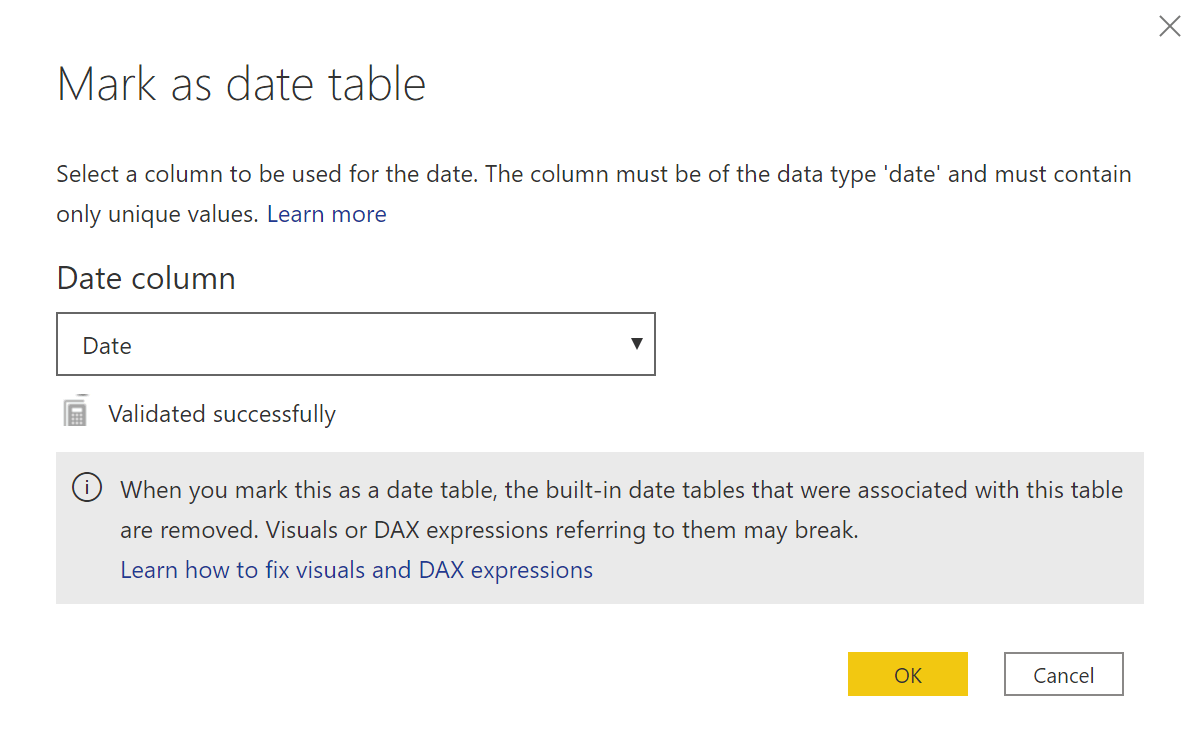Once you mark the Date table as a date table, the results become correct again. It is worth to note that the additional REMOVEFILTERS is applied only when you apply a filter on the Date[Date] column. If you move the filter to any other column, then REMOVEFILTERS is not applied to the filter context.

For example, the following measure adds a filter on the Date[Calendar Year] column. The engine only replaces the filter on the Calendar Year column, and keeps any other filter active:

```Sales 2008 =
CALCULATE (
[Sales Amount],
'Date'[Calendar Year] = "CY 2008"
)
```

The Sales 2008 measure shows the amount of sales in 2008 when the report includes dates in 2007. However, the filter on the month is not removed, so every row of the report provides the value of two different years: Sales Amount shows data from 2007, and Sales 2008 shows data of the corresponding month in 2008.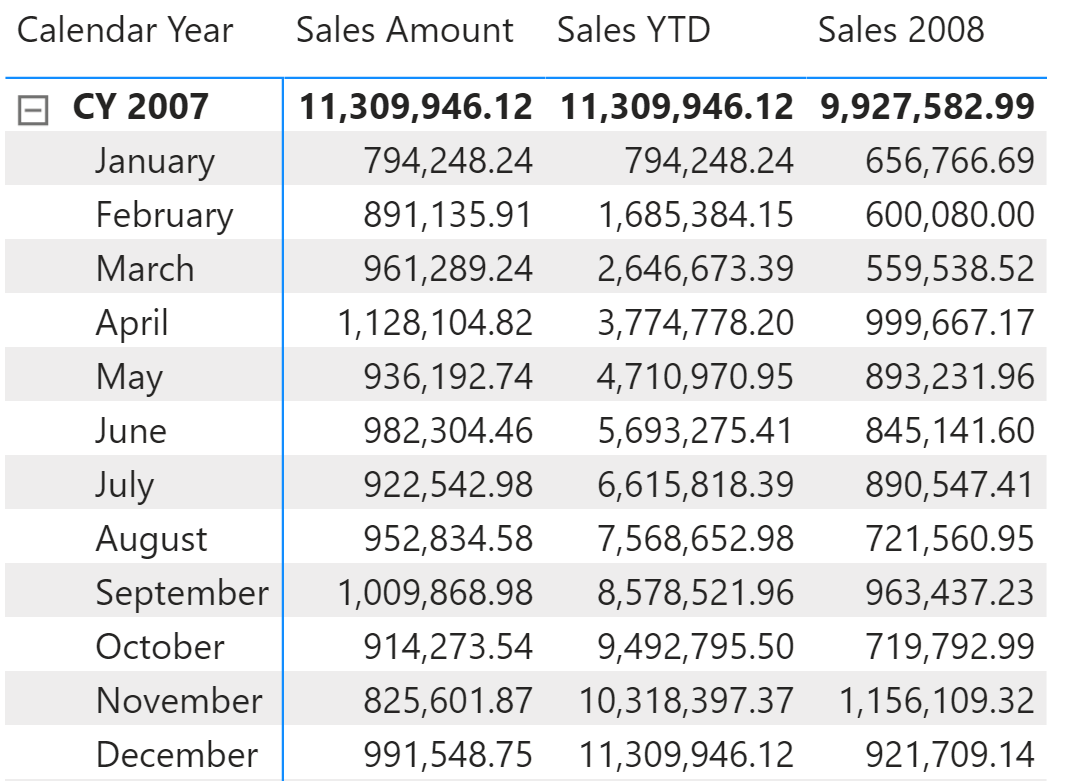If we evaluate the filter context applied on the cell of April in the report, this is the code executed for the Sales 2008 measure:

```CALCULATE (
CALCULATE (
[Sales Amount],
'Date'[Calendar Year] = "CY 2008"
),
'Date'[Calendar Year] = "CY 2007",
'Date'[Month] = "April"
)
```

The inner filter on 2008 replaces the outer filter on the Calendar Year column, without replacing the outer filter on the month. As expected, the result includes the sales in April 2008.

Now that you know what happens when you mark a table as a date table, you can conclude the following:

• If the relationship between Sales and Date is based on a column of Date data type, DAX automatically applies REMOVEFILTERS over the Date There is no need to mark the table as a date table because the behavior is implicit; still, it is a good idea to continue doing so in order to enrich the model metadata so that client tools can improve the user experience.
• If the relationship between Sales and Date is based on a column of a data type other than Date, then you must mark the table as a date table to obtain the expected behavior from time intelligence calculations.
• If you do not want to mark the table as a date table, you can obtain the same behavior by adding REMOVEFILTERS ( Date ) to every calculation that uses time intelligence functions. Nonetheless, it is quite boring to add all those REMOVEFILTERS, so marking a table as a date table is a best practice.
• You can mark as many tables as date tables as needed. DAX applies the special handling of filters to each table marked as a date table.

Marking the Date table as a date table is a best practice. If you were used to doing this in the past, you can safely continue doing it. Now you also know the reason why marking a table as a date table is good. Knowing the why is always better than blindly following a rule. The results are the same, but this awareness can be invaluable to troubleshoot an incorrect result.

DATESYTDContext transition

Returns a set of dates in the year up to the last date visible in the filter context.

`DATESYTD ( <Dates> [, <YearEndDate>] )`

REMOVEFILTERSCALCULATE modifier

Clear filters from the specified tables or columns.

`REMOVEFILTERS ( [<TableNameOrColumnName>] [, <ColumnName> [, <ColumnName> [, … ] ] ] )`